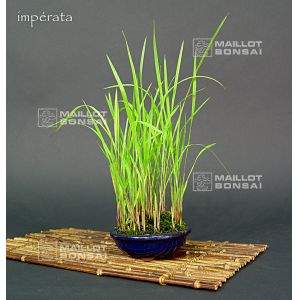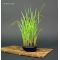##### The Japanese Bonsai specialist
Direct order Contact Help / Services Newsletter# Imperata cylindrica red grass

Chigaya kusaref. : 7259

5,40

This item is temporarily unavailableNotify availability

###### Description

Height: + - 150 mm. Delivered in a plastic pot. Bamboo mat not included. Imperata cylindrica is favoured for its fiery blood-red tips in autumn. In winter the straw turns brown while in summer the ornamental green grass features silver grey flowers. In Japanese it's called Chigaya Kusa.

#cylindrica 4 #imperata 3.8 #grass 3.5 #kusamono 2.8 #plants 2.6 #small 2.5 #ornamental 2 #delivered 1.9 #favoured 1.8 #features 1.8

Formule
(( ROUND((CHAR_LENGTH(b.article_nom)-CHAR_LENGTH(REPLACE(b.article_nom, 'cylindrica', '')))/LENGTH('cylindrica')) + ROUND((CHAR_LENGTH(b.article_description)-CHAR_LENGTH(REPLACE(b.article_description, 'cylindrica', '')))/LENGTH('cylindrica')) ) * 4) + (( ROUND((CHAR_LENGTH(b.article_nom)-CHAR_LENGTH(REPLACE(b.article_nom, 'imperata', '')))/LENGTH('imperata')) + ROUND((CHAR_LENGTH(b.article_description)-CHAR_LENGTH(REPLACE(b.article_description, 'imperata', '')))/LENGTH('imperata')) ) * 3.8) + (( ROUND((CHAR_LENGTH(b.article_nom)-CHAR_LENGTH(REPLACE(b.article_nom, 'grass', '')))/LENGTH('grass')) + ROUND((CHAR_LENGTH(b.article_description)-CHAR_LENGTH(REPLACE(b.article_description, 'grass', '')))/LENGTH('grass')) ) * 3.5) + (( ROUND((CHAR_LENGTH(b.article_nom)-CHAR_LENGTH(REPLACE(b.article_nom, 'ornamental', '')))/LENGTH('ornamental')) + ROUND((CHAR_LENGTH(b.article_description)-CHAR_LENGTH(REPLACE(b.article_description, 'ornamental', '')))/LENGTH('ornamental')) ) * 2) + (( ROUND((CHAR_LENGTH(b.article_nom)-CHAR_LENGTH(REPLACE(b.article_nom, 'delivered', '')))/LENGTH('delivered')) + ROUND((CHAR_LENGTH(b.article_description)-CHAR_LENGTH(REPLACE(b.article_description, 'delivered', '')))/LENGTH('delivered')) ) * 1.9) + (( ROUND((CHAR_LENGTH(b.article_nom)-CHAR_LENGTH(REPLACE(b.article_nom, 'favoured', '')))/LENGTH('favoured')) + ROUND((CHAR_LENGTH(b.article_description)-CHAR_LENGTH(REPLACE(b.article_description, 'favoured', '')))/LENGTH('favoured')) ) * 1.8) + (( ROUND((CHAR_LENGTH(b.article_nom)-CHAR_LENGTH(REPLACE(b.article_nom, 'features', '')))/LENGTH('features')) + ROUND((CHAR_LENGTH(b.article_description)-CHAR_LENGTH(REPLACE(b.article_description, 'features', '')))/LENGTH('features')) ) * 1.8) + (( ROUND((CHAR_LENGTH(b.article_nom)-CHAR_LENGTH(REPLACE(b.article_nom, 'japanese', '')))/LENGTH('japanese')) + ROUND((CHAR_LENGTH(b.article_description)-CHAR_LENGTH(REPLACE(b.article_description, 'japanese', '')))/LENGTH('japanese')) ) * 1.8) + (( ROUND((CHAR_LENGTH(b.article_nom)-CHAR_LENGTH(REPLACE(b.article_nom, 'included', '')))/LENGTH('included')) + ROUND((CHAR_LENGTH(b.article_description)-CHAR_LENGTH(REPLACE(b.article_description, 'included', '')))/LENGTH('included')) ) * 1.8) + (( ROUND((CHAR_LENGTH(b.article_nom)-CHAR_LENGTH(REPLACE(b.article_nom, 'plastic', '')))/LENGTH('plastic')) + ROUND((CHAR_LENGTH(b.article_description)-CHAR_LENGTH(REPLACE(b.article_description, 'plastic', '')))/LENGTH('plastic')) ) * 1.7)

## Secure payment## Delivery

Our logistic partners :04 74 55 23 48
Pépinière MAILLOT-BONSAÏ
Le Bois Frazy
01990 RELEVANT - FRANCE
on appointmentThe Japanese Bonsai specialist
If you like Maillot Bonsaï, share on  facebook

A vos côtés. Les services restent les mêmes.

La vente continue et les expéditions également.

Meilleurs vœux pour 2021.

Guy Maillot

OK